# Introduction

This vignette visualizes classification results from k-nearest neighbors, using tools from the package.

``````library("classmap")
``````

# Iris data

As a first small example, we consider the Iris data. We first load the data and inspect it. We also scale the data, to make the results of the k-nearest neighbor classifier scale-invariant.

``````data(iris)
X <- iris[, 1:4]
y <- iris[, 5]
is.factor(y)
``````
``````##  TRUE
``````
``````table(y)
``````
``````## y
##     setosa versicolor  virginica
##         50         50         50
``````
``````X <- scale(X) # if you want to scale, best to do it yourself.
pairs(X, col = as.numeric(y) + 1, pch = 19)
``````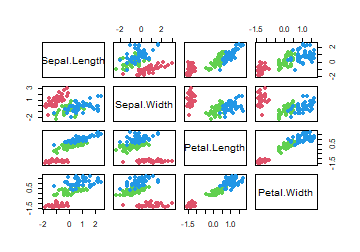``````dis <- dist(X, method = "euclidean")
``````

The VCR object for the k-nearest neighbors classifier can be constructed based on a distance matrix or the original data. We use k = 5 here:

``````vcr.train <- vcr.knn.train(dis, y, k = 5)

vcr.train <- vcr.knn.train(X, y, k = 5) # Gives the same result.
``````

Let's inspect some of the elements in the output of the vcr.train function; We first look at the prediction as integer, prediction as label, the alternative label as integer and the alternative label.

``````names(vcr.train)
``````
``````##   "yint"      "y"         "levels"    "predint"   "pred"      "altint"
##   "altlab"    "PAC"       "figparams" "fig"       "farness"   "ofarness"
##  "k"         "ktrues"    "counts"    "X"
``````
``````vcr.train\$predint
``````
``````##    1 1 1 1 1 1 1 1 1 1 1 1 1 1 1 1 1 1 1 1 1 1 1 1 1 1 1 1 1 1 1 1 1 1 1 1 1
##   1 1 1 1 1 1 1 1 1 1 1 1 1 2 2 2 2 2 2 2 2 2 2 2 2 2 2 2 2 2 2 2 2 3 2 3 2
##   2 2 2 3 2 2 2 2 2 3 2 2 2 2 2 2 2 2 2 2 2 2 2 2 2 2 3 3 3 3 3 3 2 3 3 3 3
##  3 3 3 3 3 3 3 3 2 3 3 3 3 3 3 3 3 3 3 3 3 3 2 2 3 3 3 3 3 3 3 3 3 3 3 3 3
##  3 3
``````
``````vcr.train\$pred[c(1:10, 51:60, 101:110)]
``````
``````##   "setosa"     "setosa"     "setosa"     "setosa"     "setosa"
##   "setosa"     "setosa"     "setosa"     "setosa"     "setosa"
##  "versicolor" "versicolor" "versicolor" "versicolor" "versicolor"
##  "versicolor" "versicolor" "versicolor" "versicolor" "versicolor"
##  "virginica"  "virginica"  "virginica"  "virginica"  "virginica"
##  "virginica"  "versicolor" "virginica"  "virginica"  "virginica"
``````
``````vcr.train\$altint
``````
``````##    NA NA NA NA NA NA NA NA NA NA NA NA NA NA NA NA NA NA NA NA NA NA NA NA NA
##   NA NA NA NA NA NA NA NA NA NA NA NA NA NA NA NA  2 NA NA NA NA NA NA NA NA
##   NA NA NA NA  3 NA  3 NA NA NA NA NA NA  3 NA NA NA NA  3 NA  3 NA  3 NA NA
##   NA NA  3 NA NA NA NA NA  3 NA NA NA  3 NA NA NA NA NA NA NA NA NA NA NA NA
##  NA  2 NA NA NA NA  2 NA  2 NA NA NA NA  2 NA NA  2 NA NA  2 NA NA NA  2 NA
##  NA  2  2 NA  2 NA NA NA  2  2 NA NA  2  2 NA NA NA  2 NA NA NA  2 NA NA  2
``````

The NA's stem from k-neighborhoods in which all members are from the given class. In such cases there is no alternative class.

``````vcr.train\$altlab  # has NA's for the same cases:
``````
``````##    NA           NA           NA           NA           NA
##    NA           NA           NA           NA           NA
##   NA           NA           NA           NA           NA
##   NA           NA           NA           NA           NA
##   NA           NA           NA           NA           NA
##   NA           NA           NA           NA           NA
##   NA           NA           NA           NA           NA
##   NA           NA           NA           NA           NA
##   NA           "versicolor" NA           NA           NA
##   NA           NA           NA           NA           NA
##   NA           NA           NA           NA           "virginica"
##   NA           "virginica"  NA           NA           NA
##   NA           NA           NA           "virginica"  NA
##   NA           NA           NA           "virginica"  NA
##   "virginica"  NA           "virginica"  NA           NA
##   NA           NA           "virginica"  NA           NA
##   NA           NA           NA           "virginica"  NA
##   NA           NA           "virginica"  NA           NA
##   NA           NA           NA           NA           NA
##   NA           NA           NA           NA           NA
##  NA           "versicolor" NA           NA           NA
##  NA           "versicolor" NA           "versicolor" NA
##  NA           NA           NA           "versicolor" NA
##  NA           "versicolor" NA           NA           "versicolor"
##  NA           NA           NA           "versicolor" NA
##  NA           "versicolor" "versicolor" NA           "versicolor"
##  NA           NA           NA           "versicolor" "versicolor"
##  NA           NA           "versicolor" "versicolor" NA
##  NA           NA           "versicolor" NA           NA
##  NA           "versicolor" NA           NA           "versicolor"
``````

The output also contains the value of k, which was an input value, as well as the “true” value of k used for each instance, which can differ from the input value of k in case of ties:

``````vcr.train\$k
``````
``````##  5
``````
``````vcr.train\$ktrues[1:10]
``````
``````##   6 5 5 6 5 5 5 5 5 5
``````

We can also extract the probability of Alternative Class (PAC) of each object, which we will use later to construct the class map:

``````vcr.train\$PAC # length 150, all between 0 and 1, no NA's
``````
``````##    0.0 0.0 0.0 0.0 0.0 0.0 0.0 0.0 0.0 0.0 0.0 0.0 0.0 0.0 0.0 0.0 0.0 0.0
##   0.0 0.0 0.0 0.0 0.0 0.0 0.0 0.0 0.0 0.0 0.0 0.0 0.0 0.0 0.0 0.0 0.0 0.0
##   0.0 0.0 0.0 0.0 0.0 0.4 0.0 0.0 0.0 0.0 0.0 0.0 0.0 0.0 0.0 0.0 0.0 0.0
##   0.2 0.0 0.2 0.0 0.0 0.0 0.0 0.0 0.0 0.2 0.0 0.0 0.0 0.0 0.4 0.0 0.6 0.0
##   0.6 0.0 0.0 0.0 0.0 0.6 0.0 0.0 0.0 0.0 0.0 1.0 0.0 0.0 0.0 0.2 0.0 0.0
##   0.0 0.0 0.0 0.0 0.0 0.0 0.0 0.0 0.0 0.0 0.0 0.2 0.0 0.0 0.0 0.0 0.8 0.0
##  0.2 0.0 0.0 0.0 0.0 0.2 0.0 0.0 0.2 0.0 0.0 1.0 0.0 0.0 0.0 0.2 0.0 0.0
##  0.2 0.2 0.0 0.2 0.0 0.0 0.0 0.6 0.6 0.0 0.0 0.2 0.4 0.0 0.0 0.0 0.2 0.0
##  0.0 0.0 0.4 0.0 0.0 0.4
``````

The second ingredient of the class map is the farness, which is computed from the fig values defined as f(i, g)= the distance from case i to class g:

``````vcr.train\$fig[1:5, ]
``````
``````##            [,1]      [,2]      [,3]
## [1,] 0.04461133 0.9996704 0.9986414
## [2,] 0.03342043 0.9990550 0.9984272
## [3,] 0.31360938 0.9996165 0.9986936
## [4,] 0.24999968 0.9994512 0.9986348
## [5,] 0.26756761 0.9997711 0.9988206
``````

The farness of an object i is the f(i, g) to its own class:

``````vcr.train\$farness[1:5]
``````
``````##  0.04461133 0.03342043 0.31360938 0.24999968 0.26756761
``````
``````summary(vcr.train\$farness)
``````
``````##    Min. 1st Qu.  Median    Mean 3rd Qu.    Max.
## 0.01647 0.25000 0.50000 0.50560 0.76622 0.99669
``````

The “overall farness” of an object is defined as the lowest f(i, g) it has to any class g (including its own). This always exists.

``````summary(vcr.train\$ofarness)
``````
``````##    Min. 1st Qu.  Median    Mean 3rd Qu.    Max.
## 0.01647 0.25000 0.49745 0.49660 0.74040 0.99669
``````

Using confmat.vcr(), we can construct the usual confusion matrix and obtain the accuracy of the classification:

``````confmat.vcr(vcr.train, showOutliers = FALSE)
``````
``````##
## Confusion matrix:
##             predicted
## given        setosa versicolor virginica
##   setosa         50          0         0
##   versicolor      0         46         4
##   virginica       0          4        46
##
## The accuracy is 94.67%.
``````

By using showOutliers = TRUE and setting a cutoff, objects with ofarness > cutoff are flagged as outliers and shown in a separate column of the confusion matrix:

``````confmat.vcr(vcr.train, cutoff = 0.98)
``````
``````##
## Confusion matrix:
##             predicted
## given        setosa versicolor virginica outl
##   setosa         49          0         0    1
##   versicolor      0         46         4    0
##   virginica       0          4        44    2
##
## The accuracy is 94.67%.
``````

With the default cutoff = 0.99 only one object is flagged in this example:

``````confmat.vcr(vcr.train)
``````
``````##
## Confusion matrix:
##             predicted
## given        setosa versicolor virginica outl
##   setosa         49          0         0    1
##   versicolor      0         46         4    0
##   virginica       0          4        46    0
##
## The accuracy is 94.67%.
``````

Note that the accuracy is computed before any objects are flagged, so it does not depend on the cutoff. Finally, we can also show the class numbers instead of the labels in the confusion matrix. This option can be useful for long level names.

``````confmat.vcr(vcr.train, showClassNumbers = TRUE)
``````
``````##
## Confusion matrix:
##      predicted
## given  1  2  3 outl
##     1 49  0  0    1
##     2  0 46  4    0
##     3  0  4 46    0
##
## The accuracy is 94.67%.
``````

The stacked mosaic plot is a graphical representation of the confusion matrix, and can be made using the stackedplot() function. The outliers can optionally be shown as separate gray areas on top of each rectangle:

``````par(mfrow = c(1, 1))
cols <- c("red", "darkgreen", "blue")
stackedplot(vcr.train, classCols = cols, separSize = 1.5,
minSize = 1, showOutliers = FALSE, showLegend = TRUE,
main = "Stacked plot of kNN on iris data")
``````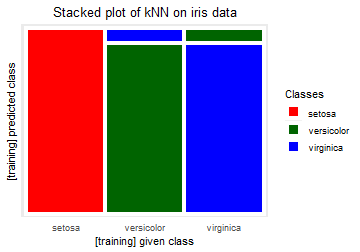``````stackedplot(vcr.train, classCols = cols, separSize = 1.5,
minSize = 1, showLegend = TRUE,
main = "Stacked plot of kNN on iris data")
``````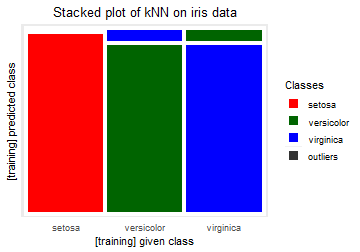``````par(oldpar)
``````

By default, no legend is added to the stacked mosaic plot, because we see the colors at the bottom of each given class anyway:

``````stplot <- stackedplot(vcr.train, classCols = cols,
separSize = 1.5, minSize = 1,
main = "Stacked plot of kNN on iris data")
stplot
``````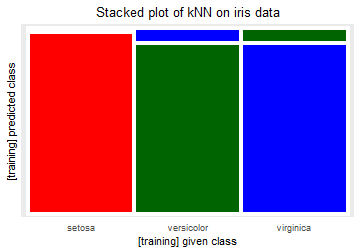We now construct the silhouette plot:

``````# pdf("Iris_kNN_silhouettes.pdf", width=5.0, height=4.6)
silplot(vcr.train, classCols = cols,
main = "Silhouette plot of kNN on iris data")
``````
``````##  classNumber classLabel classSize classAveSi
##            1     setosa        50       0.98
##            2 versicolor        50       0.84
##            3  virginica        50       0.75
``````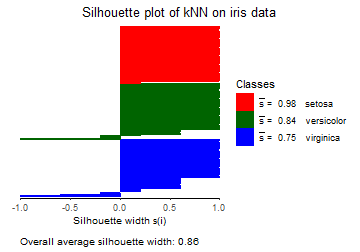``````# dev.off()
``````

Now we turn to the construction of the class maps.

For class 1, only one point has PAC > 0, around 0.4. All the others are classified perfectly:

``````classmap(vcr.train, 1, classCols = cols)
``````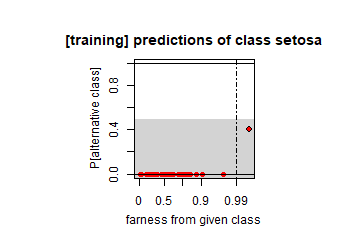Class 2 shows only 4 points with PAC > 0.5:

``````classmap(vcr.train, 2, classCols = cols)
``````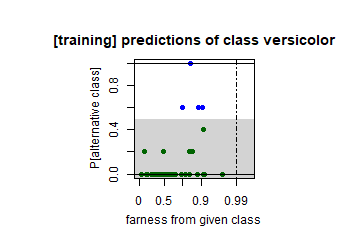Finally, class 3 also has 4 points with PAC > 0.5:

``````classmap(vcr.train, 3, classCols = cols)
``````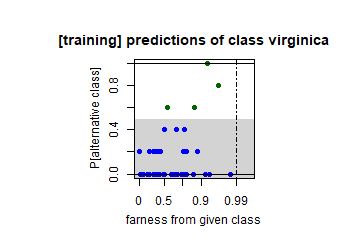To illustrate the use of new data we create a fake' dataset which is a subset of training data, where not all classes occur, and ynew has NA's. First create and inspect this test data:

``````Xnew <- X[c(1:50, 101:150),]
ynew <- y[c(1:50, 101:150)]
ynew[c(1:10, 51:60)] <- NA
pairs(X, col = as.numeric(y) + 1, pch = 19) # 3 colors
````````````pairs(Xnew, col = as.numeric(ynew) + 1, pch = 19) # only red and blue
``````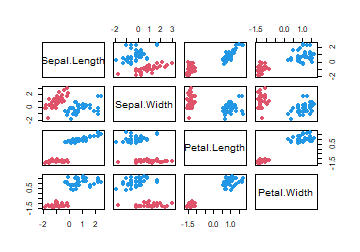Now build the VCR object on the new data, using the output vcr.train which was built on the training data.

``````vcr.test <- vcr.knn.newdata(Xnew, ynew, vcr.train, LOO = TRUE)
``````

We perform a few sanity checks by comparing the vcr object of the training data with that of the test data. Note that the following only match when LOO = TRUE since we use “leave-one-out” in training! The default is LOO = FALSE.

``````plot(vcr.test\$predint, vcr.train\$predint[c(1:50, 101:150)]); abline(0, 1)
``````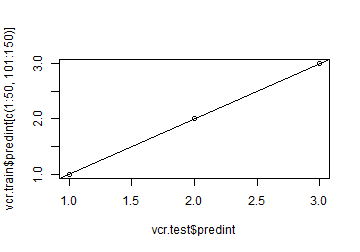``````plot(vcr.test\$altint, vcr.train\$altint[c(1:50, 101:150)]); abline(0, 1)
``````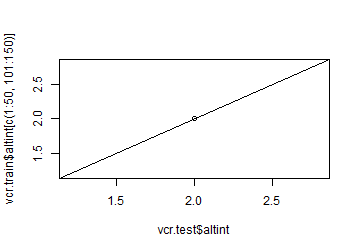``````plot(vcr.test\$PAC, vcr.train\$PAC[c(1:50, 101:150)]); abline(0, 1)
``````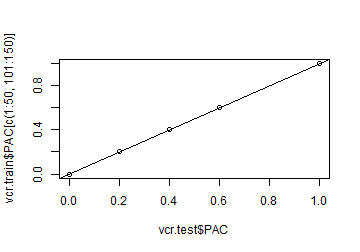``````vcr.test\$farness
``````
``````##            NA         NA         NA         NA         NA         NA
##            NA         NA         NA         NA 0.73247121 0.36589548
##   0.24999968 0.79924753 0.87396416 0.97491095 0.79924753 0.04461133
##   0.79924753 0.24610411 0.52074700 0.54514841 0.82815149 0.75324677
##   0.64247009 0.36138149 0.36138149 0.15373845 0.19064246 0.22847962
##   0.19064246 0.62411021 0.90504454 0.87228426 0.15373845 0.49806452
##   0.62411021 0.59563405 0.54175612 0.15373845 0.26756761 0.99669277
##   0.56908070 0.70411547 0.45330349 0.33045955 0.50193548 0.15373845
##   0.55447868 0.24999968         NA         NA         NA         NA
##           NA         NA         NA         NA         NA         NA
##   0.31224622 0.29161308 0.17210482 0.79288558 0.78742650 0.50317237
##   0.01647377 0.98448662 0.92264168 0.93153659 0.08394010 0.65348271
##   0.69542454 0.33736954 0.42034585 0.76741891 0.29161308 0.36680188
##   0.34391108 0.76263566 0.73701673 0.98482904 0.40090355 0.54448626
##   0.86070226 0.84818064 0.50317237 0.20998164 0.49682763 0.08394010
##   0.04045957 0.09945973 0.42645401 0.07996972 0.37628241 0.02454008
##   0.76862586 0.17210482 0.50549224 0.68050661
``````
``````plot(vcr.test\$farness, vcr.train\$farness[c(1:50, 101:150)]); abline(0, 1)
``````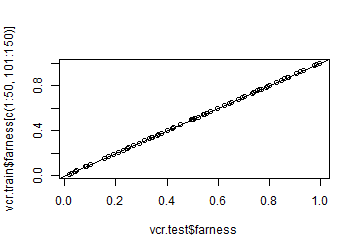``````plot(vcr.test\$fig, vcr.train\$fig[c(1:50, 101:150), ]); abline(0, 1)
``````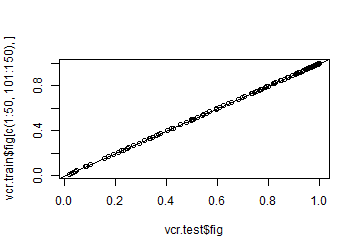``````vcr.test\$ofarness  # This does exist for every case, even if is given label is NA:
``````
``````##    0.04461133 0.03342043 0.31360938 0.24999968 0.26756761 0.73406966
##    0.77153198 0.19064246 0.81769352 0.24999968 0.73247121 0.36589548
##   0.24999968 0.79924753 0.87396416 0.97491095 0.79924753 0.04461133
##   0.79924753 0.24610411 0.52074700 0.54514841 0.82815149 0.75324677
##   0.64247009 0.36138149 0.36138149 0.15373845 0.19064246 0.22847962
##   0.19064246 0.62411021 0.90504454 0.87228426 0.15373845 0.49806452
##   0.62411021 0.59563405 0.54175612 0.15373845 0.26756761 0.99669277
##   0.56908070 0.70411547 0.45330349 0.33045955 0.50193548 0.15373845
##   0.55447868 0.24999968 0.47804488 0.42645401 0.60529406 0.36680188
##   0.22408548 0.68239080 0.96393539 0.60529406 0.87814119 0.93697386
##   0.31224622 0.29161308 0.17210482 0.79288558 0.78742650 0.50317237
##   0.01647377 0.98448662 0.92264168 0.92252602 0.08394010 0.65348271
##   0.69542454 0.33736954 0.42034585 0.76741891 0.29161308 0.36680188
##   0.34391108 0.76263566 0.73701673 0.98482904 0.40090355 0.54448626
##   0.86070226 0.84818064 0.50317237 0.20998164 0.49682763 0.08394010
##   0.04045957 0.09945973 0.42645401 0.07996972 0.37628241 0.02454008
##   0.76862586 0.17210482 0.50549224 0.68050661
``````
``````plot(vcr.test\$ofarness, vcr.train\$ofarness[c(1:50, 101:150)]); abline(0, 1)
``````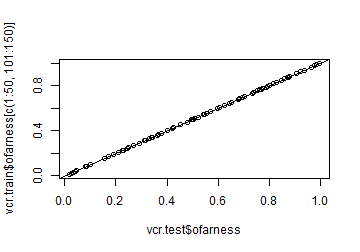We now construct and inspect the confusion matrix and the stacked mosaic plot. We plot the mosaic plot on the training data again, for comparison:

``````confmat.vcr(vcr.test)
``````
``````##
## Confusion matrix:
##            predicted
## given       setosa versicolor virginica outl
##   setosa        39          0         0    1
##   virginica      0          3        37    0
##
## The accuracy is 96.25%.
``````
``````stplot
````````````stackedplot(vcr.test, classCols = cols, separSize = 1.5, minSize = 1, main = "Stacked plot of kNN on iris subset")
``````
``````##
## Not all classes occur in these data. The classes to plot are:
##  1 3
``````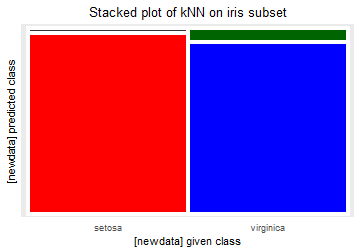And we also make the silhouette plot on the test data:

``````# pdf("Iris_test_kNN_silhouettes.pdf", width=5.0, height=4.6)
silplot(vcr.test, classCols = cols,
main = "Silhouette plot of kNN on iris subset")
``````
``````##  classNumber classLabel classSize classAveSi
##            1     setosa        40       0.98
##            3  virginica        40       0.75
``````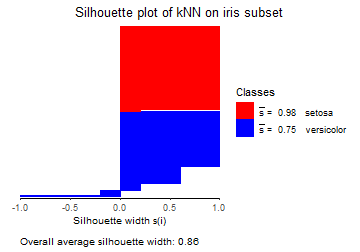``````# dev.off()
``````

For each class, we now make the class map on the test data, and compare with the class map on the training data. First for class 1:

``````classmap(vcr.train, 1, classCols = cols)
````````````classmap(vcr.test, 1, classCols = cols)
``````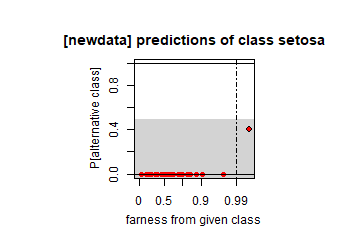Now for class 2. This throws an error on the test data, as there are no observations with the label versicolor in the test data.

``````classmap(vcr.train, 2, classCols = cols)
````````````classmap(vcr.test, 2, classCols = cols)
``````
``````## Error in classmap(vcr.test, 2, classCols = cols): Class number 2 with label versicolor has no objects to visualize.
``````
``````# Class number 2 with label versicolor has no objects to visualize.
``````

Finally for class 3. It looks the same as the class map for the training data, but there are fewer points:

``````classmap(vcr.train, 3, classCols = cols)
````````````classmap(vcr.test, 3, classCols = cols) # same, but fewer points
``````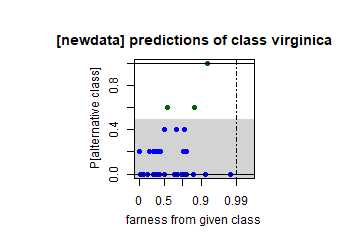# Spam data

In the example we analyze the spam data. It can be obtained via the kernlab package. It contains 4601 emails, 57 variables and a categorical variable indicating whether an email is nonspam or spam:

``````library(kernlab)
``````
``````##
## Attaching package: 'kernlab'
``````
``````## The following object is masked from 'package:ggplot2':
##
##     alpha
``````
``````#?kernlab::spam
data(spam)
``````

We now create a matrix of predictors and a response vector. Inspection shows that the predictors contain 394 duplicate rows:

``````y <- spam\$type
table(y)
``````
``````## y
## nonspam    spam
##    2788    1813
``````
``````X <- spam[, -58]
sum(duplicated(X))
``````
``````##  394
``````

Now we can construct the vcr object based on the k-nearest neighbor classifier. All knn computations will be done on scale(X), but we keep the original data X for interpreting the results.

``````vcr.obj <- vcr.knn.train(scale(X), y, k = 5)
names(vcr.obj)
``````
``````##   "yint"      "y"         "levels"    "predint"   "pred"      "altint"
##   "altlab"    "PAC"       "figparams" "fig"       "farness"   "ofarness"
##  "k"         "ktrues"    "counts"    "X"
``````
``````vcr.obj\$predint[1:100]; length(vcr.obj\$predint)
``````
``````##    2 2 2 2 2 2 2 2 2 2 2 1 2 2 2 1 2 2 2 2 1 2 2 2 2 2 2 2 2 2 2 2 2 2 1 1 2
##   2 2 2 2 2 2 2 2 2 2 2 2 2 2 2 2 2 2 2 2 1 2 2 1 1 2 1 2 2 2 2 2 2 2 2 2 2
##   2 2 1 2 2 2 2 1 2 2 1 2 2 2 2 1 2 2 2 2 2 2 2 2 2 2
``````
``````##  4601
``````
``````vcr.obj\$altint[1:100]
``````
``````##    1 1 1 1 1 1 1 1 1 1 1 1 1 1 1 1 1 1 1 1 1 1 1 1 1 1 1 1 1 1 1 1 1 1 1 1 1
##   1 1 1 1 1 1 1 1 1 1 1 1 1 1 1 1 1 1 1 1 1 1 1 1 1 1 1 1 1 1 1 1 1 1 1 1 1
##   1 1 1 1 1 1 1 1 1 1 1 1 1 1 1 1 1 1 1 1 1 1 1 1 1 1
``````
``````vcr.obj\$k
``````
``````##  5
``````
``````summary(vcr.obj\$ktrues)
``````
``````##    Min. 1st Qu.  Median    Mean 3rd Qu.    Max.
##   5.000   5.000   5.000   6.453   5.000  73.000
``````

We now inspect the confusion matrices, with and without the outliers in a separate column:

``````confmat.vcr(vcr.obj, showOutliers = FALSE)
``````
``````##
## Confusion matrix:
##          predicted
## given     nonspam spam
##   nonspam    2607  181
##   spam        228 1585
##
## The accuracy is 91.11%.
``````
``````confmat.vcr(vcr.obj)
``````
``````##
## Confusion matrix:
##          predicted
## given     nonspam spam outl
##   nonspam    2548  172   68
##   spam        221 1563   29
##
## The accuracy is 91.11%.
``````

Now construct the stacked mosaic plot. The nonspam emails get the blue color, whereas the spam class is shown in red:

``````cols <- c("deepskyblue2", "red")
# nonspam is blue, spam is red

stackedplot(vcr.obj, separSize = 1.5, minSize = 1,
classCols = cols, showOutliers = FALSE,
main = "Spam data")
``````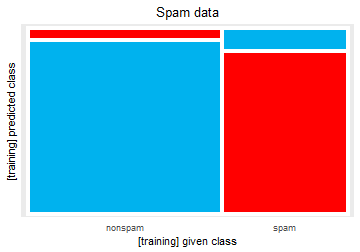``````stackedplot(vcr.obj, separSize = 1.5, minSize = 2,
classCols = cols, main = "Spam data")
``````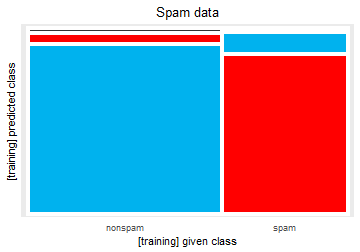We now make the silhouette plot:

``````# pdf("Spam_kNN_silhouettes.pdf", width=5.0, height=4.6)
silplot(vcr.obj, classCols = cols,
main = "Silhouette plot of kNN on spam data")
``````
``````##  classNumber classLabel classSize classAveSi
##            1    nonspam      2788       0.81
##            2       spam      1813       0.68
``````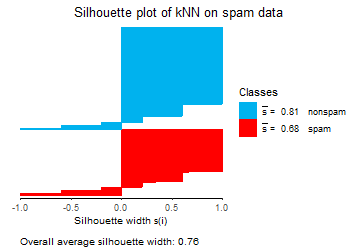``````# dev.off()
``````

Now we build the class maps. First for the nonspam messages:

``````# To identify the points that stand out:
# classmap(vcr.obj, 1, classCols = cols, identify = T)
# Press "Esc" to get out.
#
# Class map in paper:
# pdf("Spamdata_classmap_ham.pdf")
par(mar = c(3.5, 4.3, 2.0, 0.2))
coords <- classmap(vcr.obj, 1, classCols = cols,
main = "predictions of non-spam mails",
cex = 1.5, cex.lab = 1.5, cex.axis = 1.5,
cex.main = 1.5, maxfactor = 1.03)
# From identify = TRUE above we can mark points:
indstomark <- c(202, 1434, 1596, 2651, 1576, 1804)
labs  <- letters[1:length(indstomark)]
xvals <- coords[indstomark, 1] +
c(0, 0.125, 0.125, 0.125, 0.125,0.0625) # visual finetuning
yvals <- coords[indstomark, 2] +
c(-0.03, 0, 0, 0, 0, 0.03)
text(x = xvals, y = yvals, labels = labs, cex = 1.5)
legend("topleft", fill = cols,
legend = c("ham", "spam"), cex = 1,
ncol = 1, bg = "white")
``````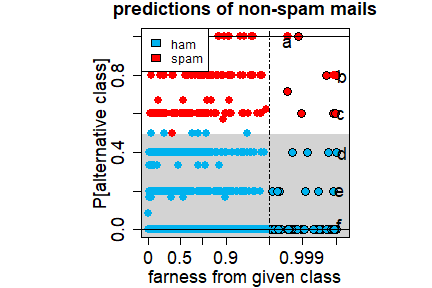``````# dev.off()
par(oldpar)
# To interpret the marked points:
# markInds = which(y == "nonspam")[indstomark]
# X[markInds, ]
``````

Now for the spam messages:

``````#
# To identify the points that stand out:
# classmap(vcr.obj, 2, classCols = cols, identify = TRUE)
# Press "Esc" to get out.
#
# Class map in paper:
# pdf("Spamdata_classmap_spam.pdf")
par(mar = c(3.5, 4.3, 2.0, 0.2))
coords <- classmap(vcr.obj, 2, classCols = cols,
main = "predictions of spam mails",
cex = 1.5, cex.lab = 1.5, cex.axis = 1.5,
cex.main = 1.5, maxfactor = 1.03)
indstomark <- c(1306, 1294, 1754, 177)
labs  <- letters[6 + (1:length(indstomark))]
xvals <- coords[indstomark, 1] + c(0.1, 0.1, 0.1, 0.1)
yvals <- coords[indstomark, 2] + c(-0.03, 0, 0, 0.03)
text(x = xvals, y = yvals, labels = labs, cex = 1.5)
legend("topleft", fill = cols,
legend = c("ham", "spam"), cex = 1,
ncol = 1, bg = "white")
``````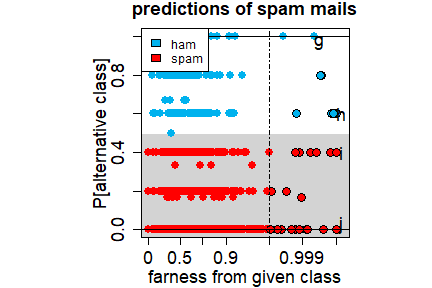``````# dev.off()
par(oldpar)
``````